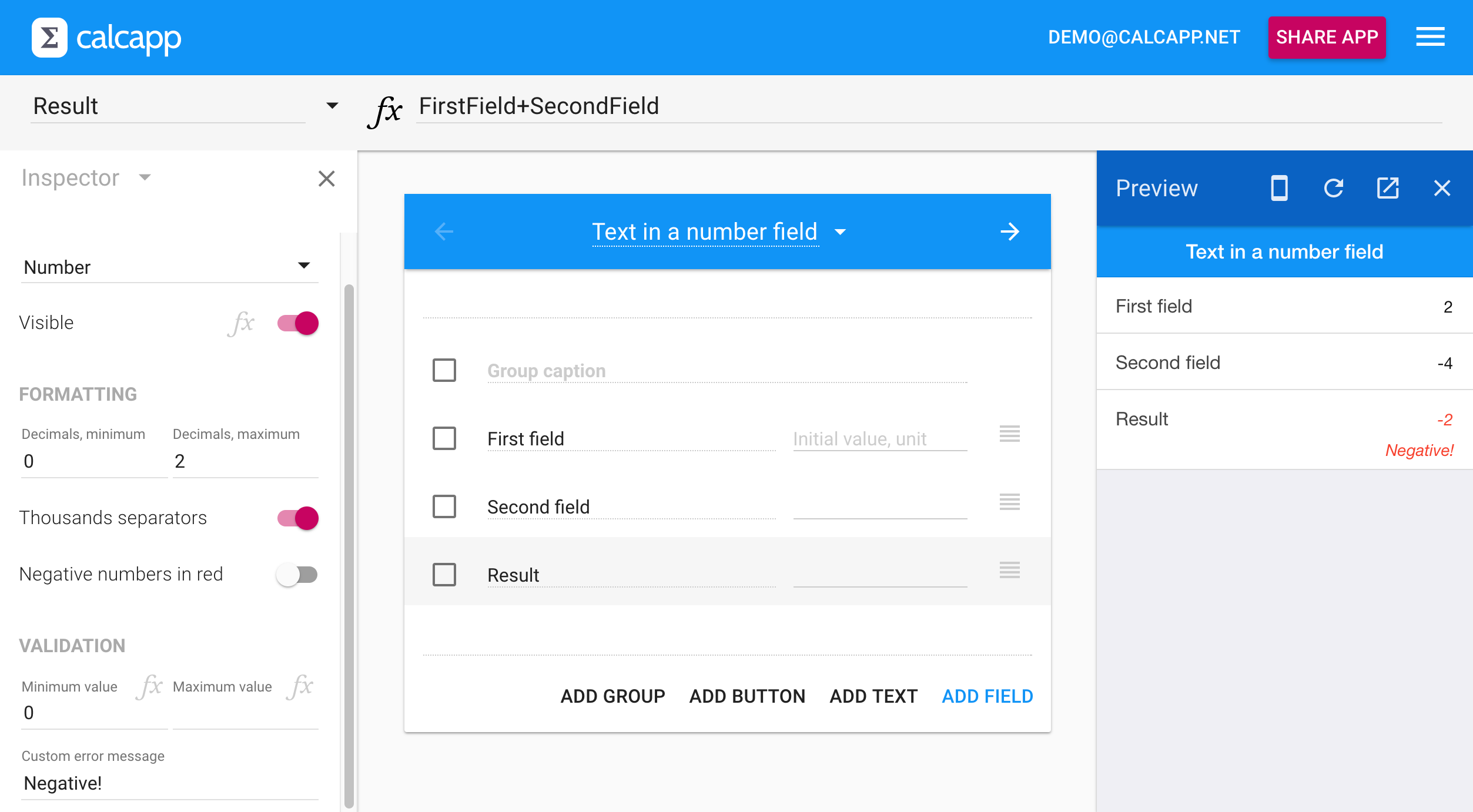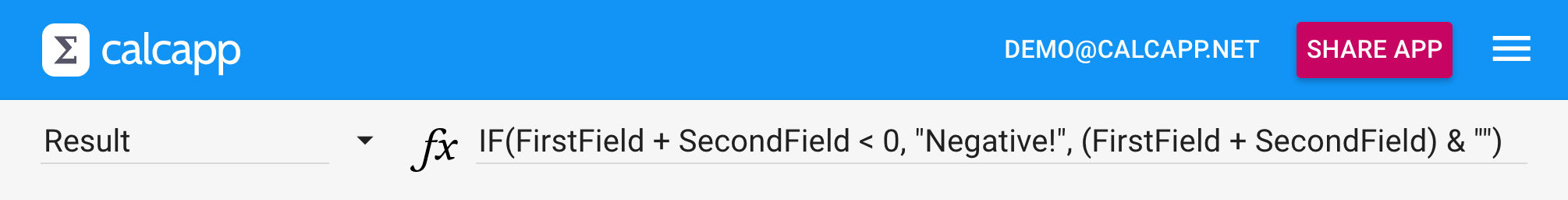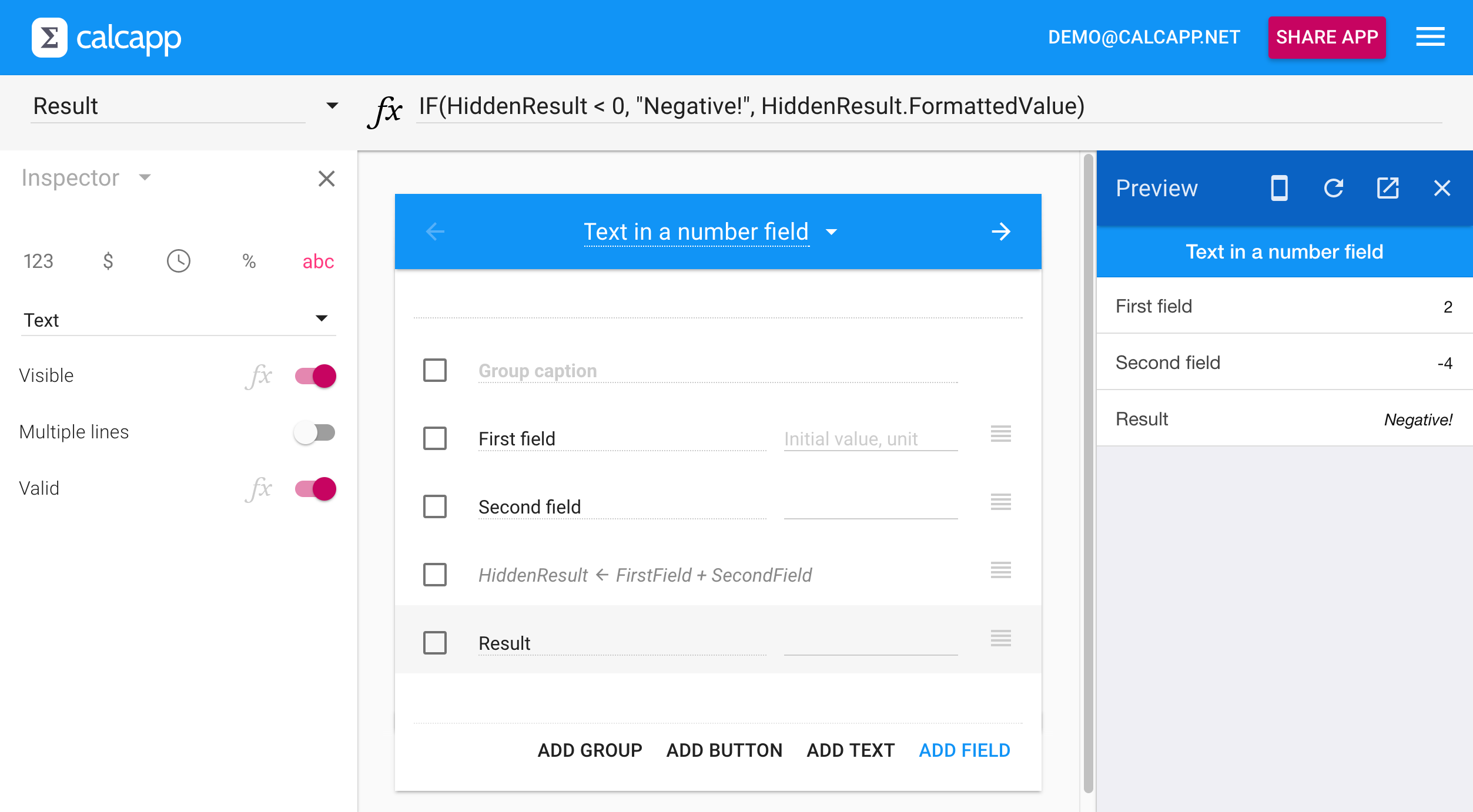• Learn
• Blog
• Pricing

# Tip: Present text in number fields

You can use data validation to present an error message alongside an output number field. Sometimes you want to display the message in the field itself, though. This blog post shows you two ways to accomplish this.

If the value of a number field is off, we suggest that you use the data validation feature to display an error message below the field in red. This works well for both input fields and output fields:Sometimes, what you really want for an output field is to present text in it, instead of presenting a potentially erroneous number alongside an error message. Perhaps it’s important to you that an erroneous value is never presented, not even with an error message. This is fully achievable with Calcapp, but it’s slightly more involved than it would have been with a traditional spreadsheet.

With a spreadsheet, you could use a formula like the following:

IF(A1 + A2 < 0, "Negative!", A1 + A2)

The cell would display the sum of the A1 and A2 cells if the sum is positive, and the text string “Negative!” if the sum is negative. You might expect this to work equally well with Calcapp, but you’re in fact presented with this error message:Like a spreadsheet cell, a field in Calcapp can only contain a number, a logical value (TRUE or FALSE) or a text string. Unlike a spreadsheet, this is something you need to determine when you design your app — a single field can’t display either a number or a text string, depending on what a formula evaluates to. This is normally a good thing, because it helps you catch errors earlier, but does make this particular situation somewhat harder to handle. (Programmers call this behavior static typing.)

You can easily solve this problem by changing the field so that it’s a text field and then ensuring that both parameters to the IF formula function are text strings. An easy way to convert a number into a text string is to concatenate (join together) a number with the empty text string (`& ""` at the end of the formula):

IF(FirstField + SecondField < 0, "Negative!", (FirstField + SecondField) & "")

As you can see, the error messsage is gone:You could also use the CONCATENATE function, which you can view as a way to convert any value to a text string:

IF(FirstField + SecondField < 0, "Negative!", CONCATENATE(FirstField + SecondField))

While that will work, we don’t actually suggest that you use this method. First, you need to repeat the entire calculation twice in your formula. Second, you have little control over how the calculated result is converted to a text string (how it’s formatted). For instance, you may want to present the value with thousands separators or with a certain number of decimal places.

We suggest that you add a hidden field instead, whose formula contains the actual calculation, and refer to its formatted value property from the field which is presented to the user. The hidden field should be a number field (enabling you to detail exactly how you want the number to be formatted), while the result field should be a text field.

The hidden field (named HiddenResult) contains only the calculation:

FirstField + SecondField

The formula for the visible result field refers to the value of the HiddenResult field when checking what text string to display, and if the value isn’t negative, refers to its formatted value:

IF(HiddenResult < 0, "Negative!", HiddenResult.FormattedValue)

Here’s the complete Calcapp Creator workspace, including a preview of the finished app. As you can see, the error message “Negative!” is now displayed in the field itself: